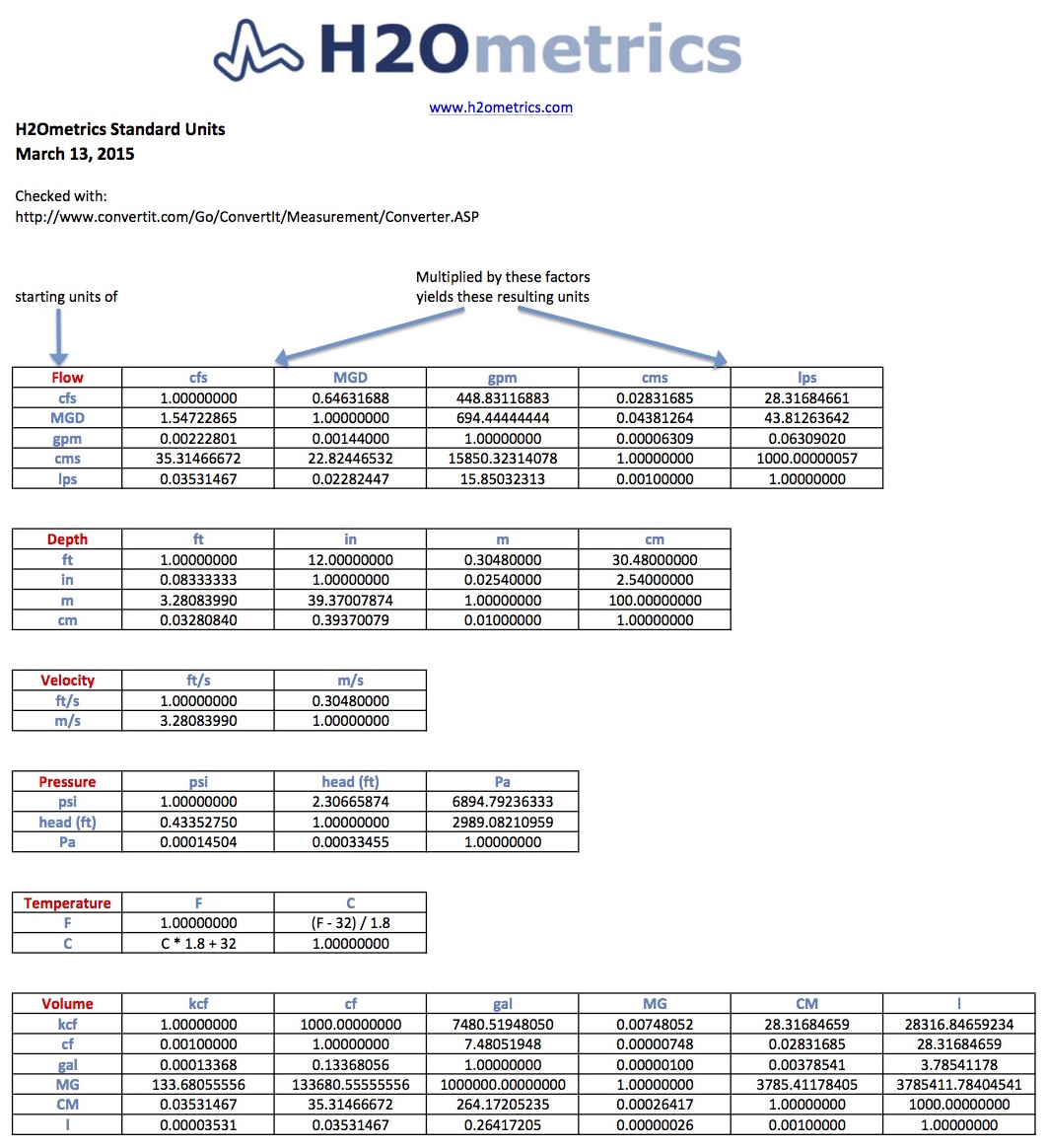Select Page

H2Ometrics uses several standard units for measuring flow, depth, velocity, pressure, temperature and volume. I thought I would share the conversions between the units in this post. The conversion table at the bottom of this post is a good reference on converting common units in general, so feel free to download and use it.

#### Standard Units Conversion Table – Free PDF

Also, we are adding new sensors types and application to H2Ometrics all the time, so if you have an application with a measurement or units not shown, just contact us and we can incorporate a new units type for your application. Here is a list of the standard units and their abbreviations:

Flow

• cfs – cubic feet per second
• MGD – millions of gallons per day
• gpm – gallons per minute
• cms – cubic meters per second
• lps – liters per second

Depth

• ft – feet
• in – inches
• m – meters
• cm – centimeters

Velocity

• ft/s – feet per second
• m/s – meters per second

Pressure

• psi – pounds per square feet
• Pa – Pascals

Temperature

• F – Fahrenheit
• C – Celsius

Volume

• kcf – thousands of cubic feet
• cf – cubic feet
• gal – gallons
• MG – millions of gallons
• CM – cubic meters
• l – liters